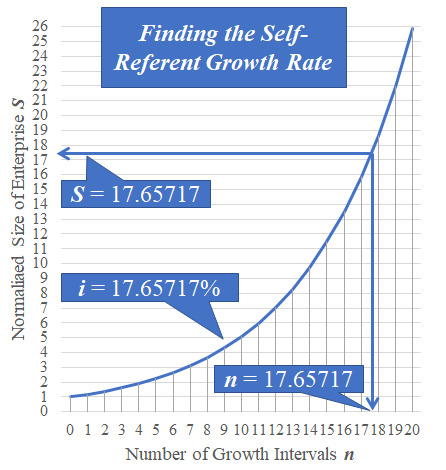his graph illustrates the derivation of an entry in the Self-Reference Collection...

 An enterprise growing at the rate of exactly 17.65717% per year will become exactly 17.65717 times larger in exactly... 17 years, seven months, 29 days, and 21 hours ...which is exactly 17.65717 years.

...and sophisticated solvers will recall that the equation S = (1+ i /100)n applies, which most often makes the relationship among the variables irrational even for integer values of S, i, and n.  Such is the inconvenience of 'exponentials' as was seen by solvers of Rational Roots.

Doubling-Time applies a most convenient formula n = 72 / i, which is well known to  economists and futurists who need to make comparisons involving growth rates.
• The formula n = 72 / i answers the question, How many growth intervals n (in years or months) are required for an 'enterprise' (or volume or budget or demand or pollution or...) that is growing at a given rate i % per growth interval to double in size S = 2?
• The formula can be turned around the other way: i = 72 / n to answer the question, How fast does an enterprise need to grow (i %) to double its size in n growth intervals?
Inasmuch as 72 is divisible by 10 integers (2, 3, 4, 6, 8, 9, 12, 18, 24, 36), the formula seldom needs a calculator.  However, as solvers of Easy as Pi know, such an approximation is not perfect.

 What error in approximation results from using the Doubling-Time formula?